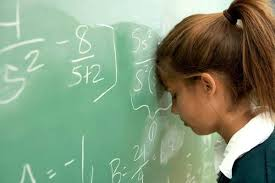Perfect SquaresSergei chooses two different natural numbers $a$ and $b$. He writes four numbers in a notebook: $a$, $a+2$, $b$ and $b+2$.

He then writes all six pairwise products of the numbers of notebook on the blackboard.

What is the maximum number of perfect squares on the blackboard?

Assumption: Natural numbers don't include zero.

×